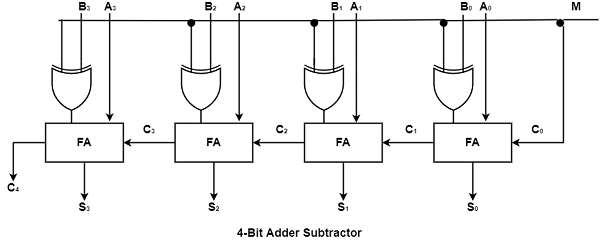# What is Binary Adder-Subtractor in Computer Architecture?

Computer ArchitectureComputer ScienceNetwork

The subtraction of binary numbers can be completed effectively by creating the 2's complement of addend bits and inserting it to the augend bits. The 2's complement can be acquired by taking the 1's complement and inserting one to the least significant pair of bits.

The 1's complement can be executed with inverters and one can be inserted into the sum by the input carry. The addition and subtraction operations can be consolidated into one joint circuit by containing an exclusive-OR gate with each full adder. A 4-bit adder-subtractor circuit is demonstrated in the figure.The mode input M regulates the operation. When M = 0 the circuit is an adder and when M = 1 the circuit turns into a subtractor. Each exclusive-OR gate receives input M and one of the inputs of B. When M = 0, we have B $\oplus$ 0 = B.

The full-adders receive the value of B, the input carry is O, and the circuit executes A plus B. When M = 1, we have B $\oplus$ 1 = B' and C0 = 1. The B inputs are all complemented and a 1 is inserted by the input carry.

The circuit operates A plus the2's complement of B. For unsigned numbers, this provides A - B if A \geq B or the 2's complement of (B - A) if A < B. For signed numbers, the result is A - B supported that there is no overflow.